# SAT II Math I : Slope

## Example Questions

### Example Question #1 : Slope

What is the slope of the line depicted by this equation?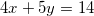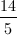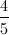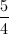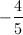Explanation:

This equation is written in standard form, that is,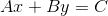where the slope is equal to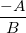.In this instance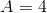and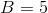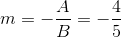This question can also be solved by converting the slope-intercept form: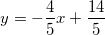.

### Example Question #2 : Slope

Find the slope of the line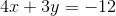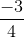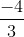Explanation:

To find the slope of any line, we must get the equation into the formwhere m is the slope and b is the y-intercept.

To manipulate our equation into this form, we must solve for y. First, we must move the x term to the right side of our equation by subtracting it from both sides.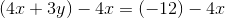To isolate y, we now must divide each side by 3.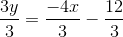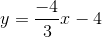Now our equation is in the desired form. The coefficient of our x term is our slope,. Therefore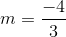### Example Question #3 : Slope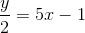What is the slope of the function above?Explanation:

First you must get the formula into slope-intercept form which means havingby itself,whereis the slope.

You must multiple both sides byto get,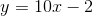.

The slope is the value being multiplied by thevariable, so our slope is.

### Example Question #4 : Slope

Find the slope of the following equation: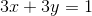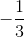Explanation:

In order to find the slope, we will need to rearrange the equation so that it is in slope-intercept form.

Subtracton both sides.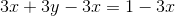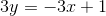Divide by three on both sides.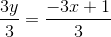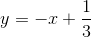This equation is now in the form of, whereis the slope.

The slope is.

### Example Question #5 : Slope

What is the slope of the following equation?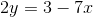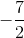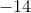Explanation:

The given equation will need to be rewritten in slope intercept format.Divide by two on both sides.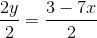Rearrange the right side by order of powers.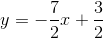The slope can be seen asThe answer is:### Example Question #1 : Slope

What is the slope of the given equation?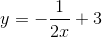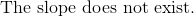Explanation:

The slope in a linear equation is defined as.

The x-variable exists in the denominator, which refers to the parent function of: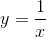This function is not linear, and will have changing slope along its domain.

The answer is: